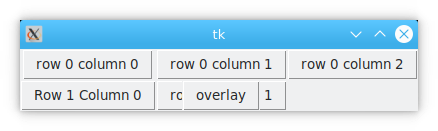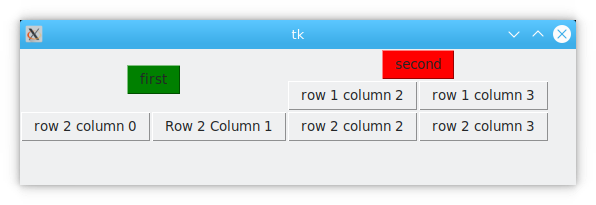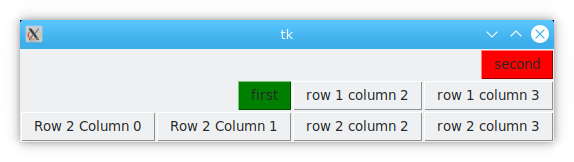# tkinter grid

The tkinter `grid()` method as how you need to place containers on top of your window, mainly by dividing the window into rows and columns of Grid. It is similar to excel, rows and columns. A little difference is that the sizes can vary unlike excel.

The grid method can be applied to all containers within Tkinter. In this article you learn how to use the tkinter grid with Python.

## tkinter grid

### row,column

The row parameter indicates the number of rows (from 0) on which the container will be placed in the window partition.

column indicates the number of columns (from 0) in which the container will be placed in the window partition.

(a) Row is a relatively worthwhile concept.

Repeated placements in the same row and column will overlap the display, with the latter overlaying the first.

``````import tkinter as tk

window = tk.Tk()
#window.geometry("300x300")
tk.Button(window,text="row 0 column 0").grid(row=0, column=0)
tk.Button(window,text="row 0 column 1").grid(row=0, column=1)
tk.Button(window,text="row 0 column 2").grid(row=0, column=5) #Here the column is set to 5, but it will still be placed in column=2. place
tk.Button(window,text="Row 1 Column 0").grid(row=1, column=0)
tk.Button(window,text="row 1 column 1").grid(row=1, column=1)
tk.Button(window,text="overlay").grid(row=1, column=1) #Place two different containers in the same location on top of each other.
window.mainloop()``````

result### rowspan, columnspan

These 2 parameters are used in conjunction with row and column, which means the row or column of the corresponding column cell will be combined into one.

``````import tkinter as tk

window = tk.Tk()
window.geometry("300x300")
tk.Button(window,text="first",bg="green")
tk.Button(window,text="first",bg="green").grid(row=0,column=0,rowspan=2,columnspan=2)
tk.Button(window,text="second",bg="red").grid( row=0,column=2,columnspan=2)
tk.Button(window,text="row 1 column 2").grid(row=1, column=2)
tk.Button(window,text="row 1 column 3").grid(row=1, column=3)
tk.Button(window,text="row 2 column 0").grid(row=2, column=0)
tk.Button(window,text="Row 2 Column 1").grid(row=2, column=1)
tk.Button(window,text="row 2 column 2").grid(row=2, column=2)
tk.Button(window,text="row 2 column 3").grid(row=2, column=3)
window.mainloop()   ``````

The results are as follows.### sticky

Indicates the alignment of the tkinter attribute within the corresponding row and column when placed.
The sticky attribute has n,s,e,w,ne,nw,se,sw parameters optional, the default centered

``````import tkinter as tk

window = tk.Tk()
#window.geometry("300x300")
tk.Button(window,text="first",bg="green")
tk.Button(window,text="first",bg="green").grid(row=0,column=0,rowspan=2,columnspan=2, sticky="se")
tk.Button(window,text="second",bg="red").grid( row=0,column=2,columnspan=2,sticky="e")
tk.Button(window,text="row 1 column 2").grid(row=1, column=2)
tk.Button(window,text="row 1 column 3").grid(row=1, column=3)
tk.Button(window,text="Row 2 Column 0").grid(row=2, column=0)
tk.Button(window,text="Row 2 Column 1").grid(row=2, column=1)
tk.Button(window,text="row 2 column 2").grid(row=2, column=2)
tk.Button(window,text="row 2 column 3").grid(row=2, column=3)
window.mainloop()``````

The results are as follows.